February 23, 2016

## Funes: Someone with perfect memory

For nineteen years he had lived as one in a dream:

• he looked without seeing, listened without hearing, forgetting everything, almost everything.

When he fell, he became unconscious; when he came to, the present was almost intolerable in its richness and sharpness

## He can see and remember everything

We, at one glance, can perceive three glasses on a table

Funes, all the leaves and tendrils and fruit that make up a grapevine

He knew by heart the forms of the southern clouds at dawn

and could compare them in his memory with the mottled streaks on a book in Spanish binding he had only seen once

## To think is to forget differences

With no effort, he had learned English, French, Portuguese and Latin.

I suspect, however, that he was not very capable of thought.

To think is to forget differences, generalize, make abstractions.

In the teeming word of Funes, there were only details, almost immediate in their presence.

## Funes remembers but does not understand

• He was almost incapable of ideas in a general, Platonic sort
• Not only was it difficult for him to comprehend that the generic symbol dog embrace so many unlike individuals of diverse size and form
• it bothered him that the dog at 3:14 (seen from the side) should have the same name as the dog at 3:15 (seen from the front).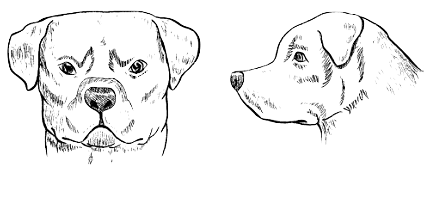## To think is to generalize, make abstractions

• Funes was not very capable of thought.
• To think is to forget differences, generalize, make abstractions.

Computers have very good memory, like Funes

## Platonic Idealism

An Idea is the essence of an object

• It defines the kind of a thing
• Ideal things are aspatial and atemporal
• they exist independent of time and space
• for example: geometric figures
• The material things we experience are shadow of the Idea

## Allegory of the Cave

### Plato’s version of The Matrix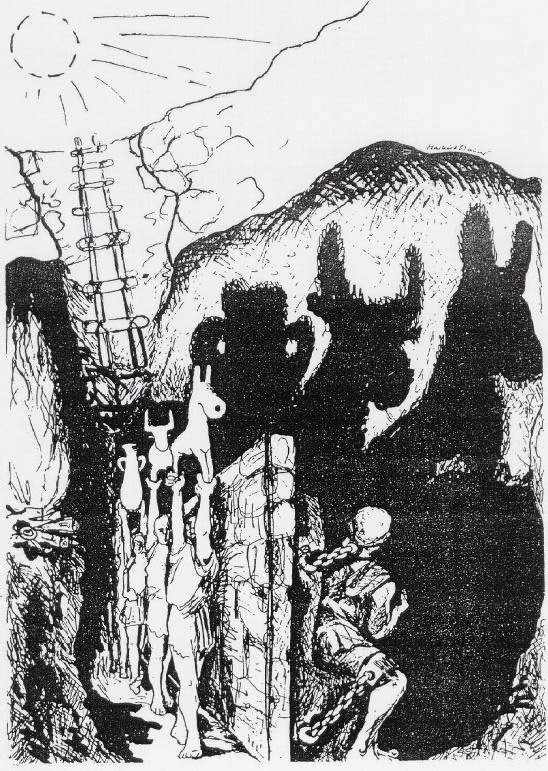## We see the same thing from any side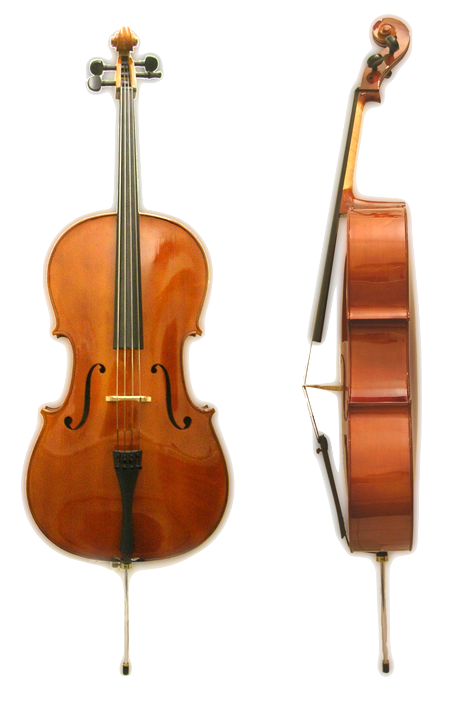## We see the same person from any side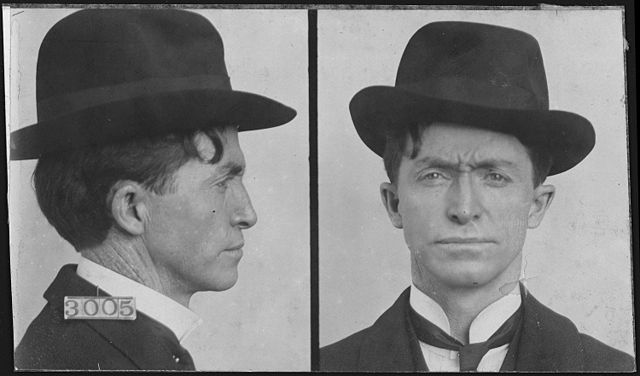## And at any age## Same essence through time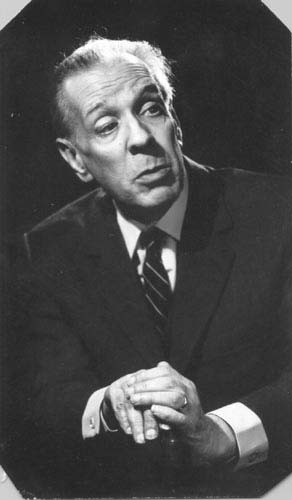## Forgetting more we see classes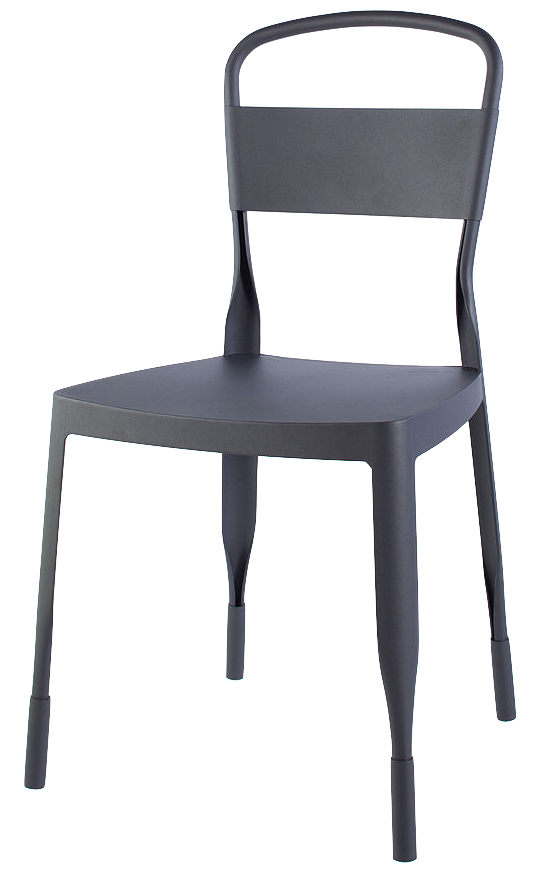## Classes: defined by a pattern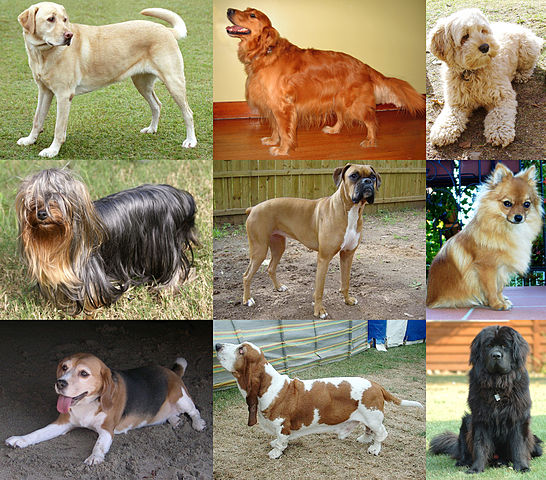## We easily recognize humans

### Our brains are very good at finding some patterns## Sometimes we overgeneralize 🙂

### Pareidolia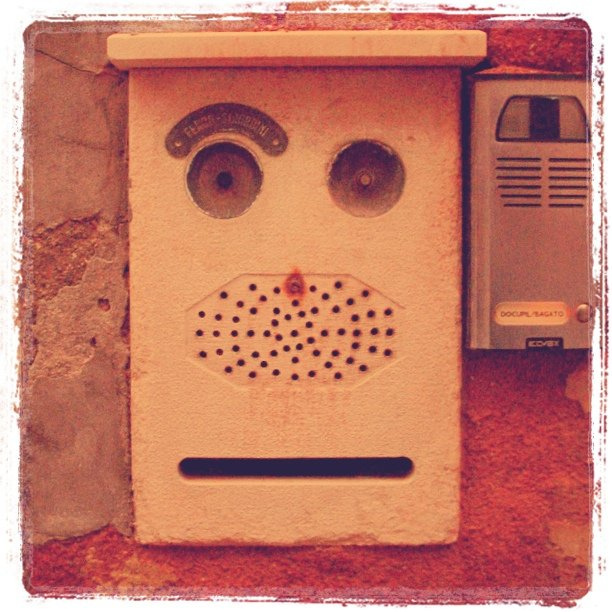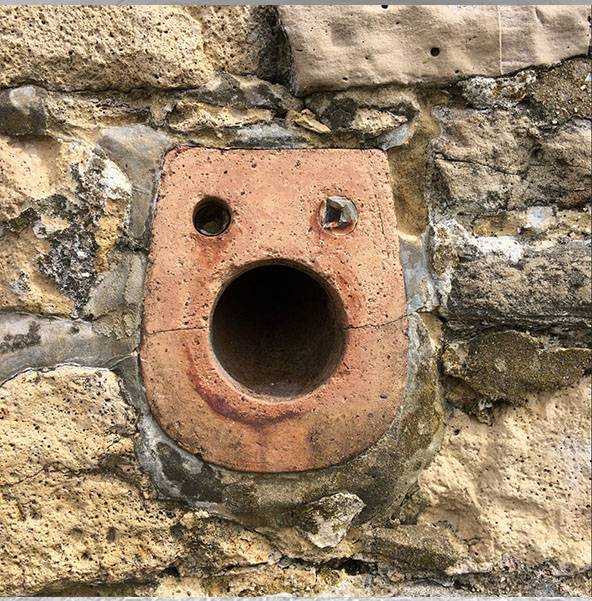## Forgetting more: higher classes

### Animals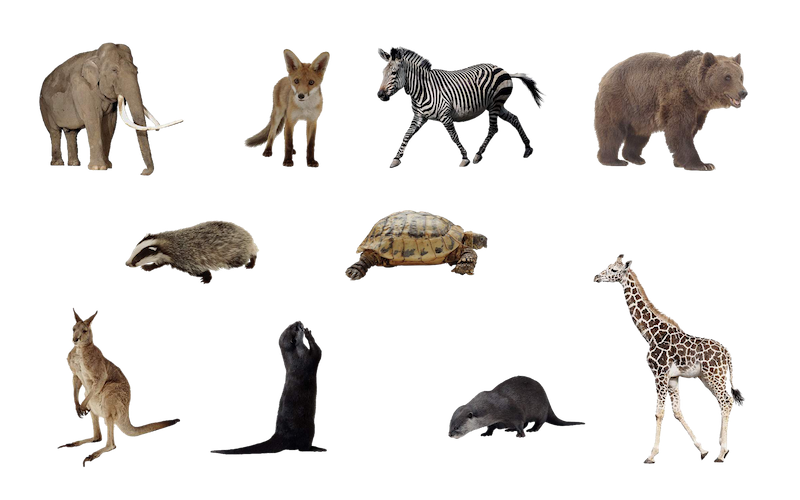## Grouping similars together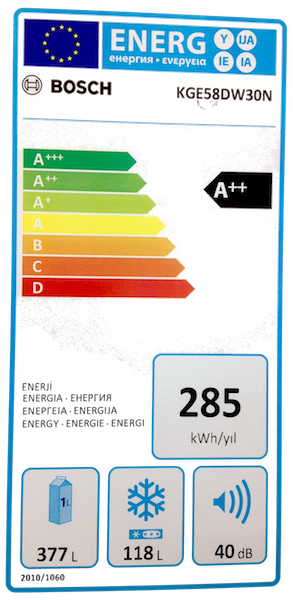## Language: forget details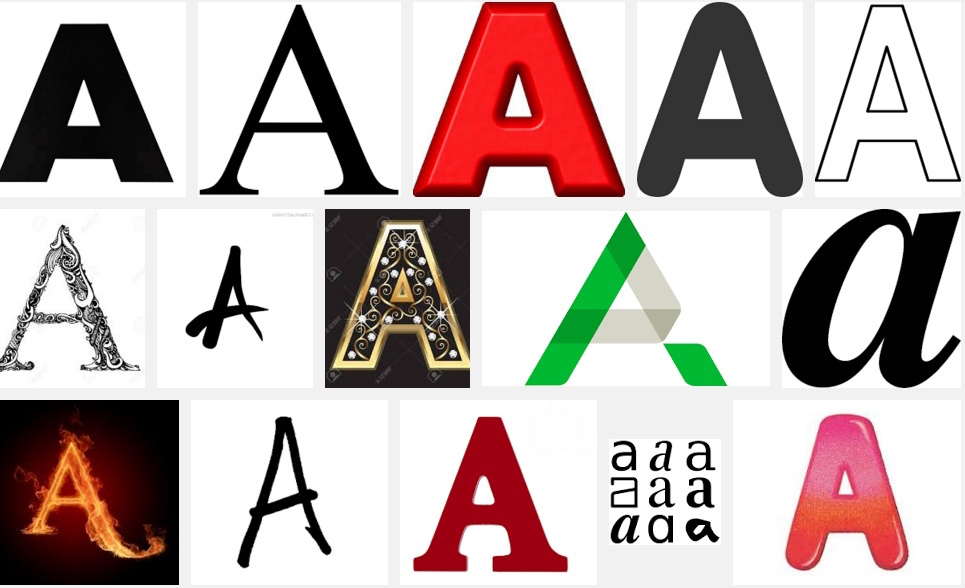## Forgetting even more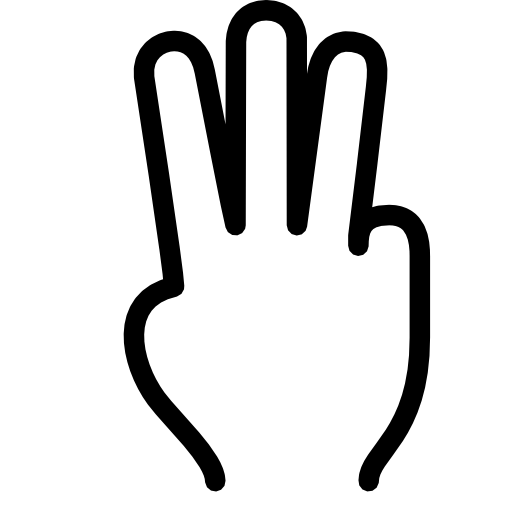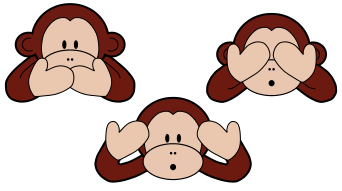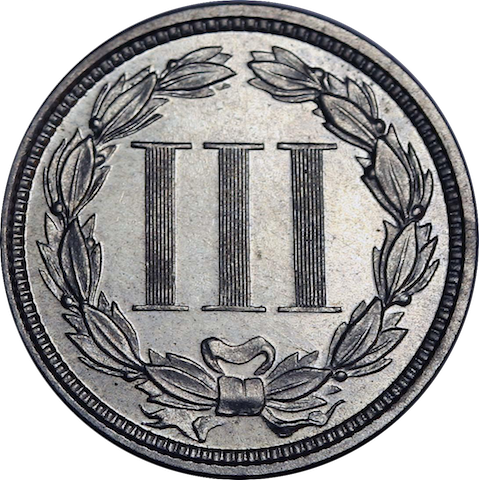## Numbers are abstractions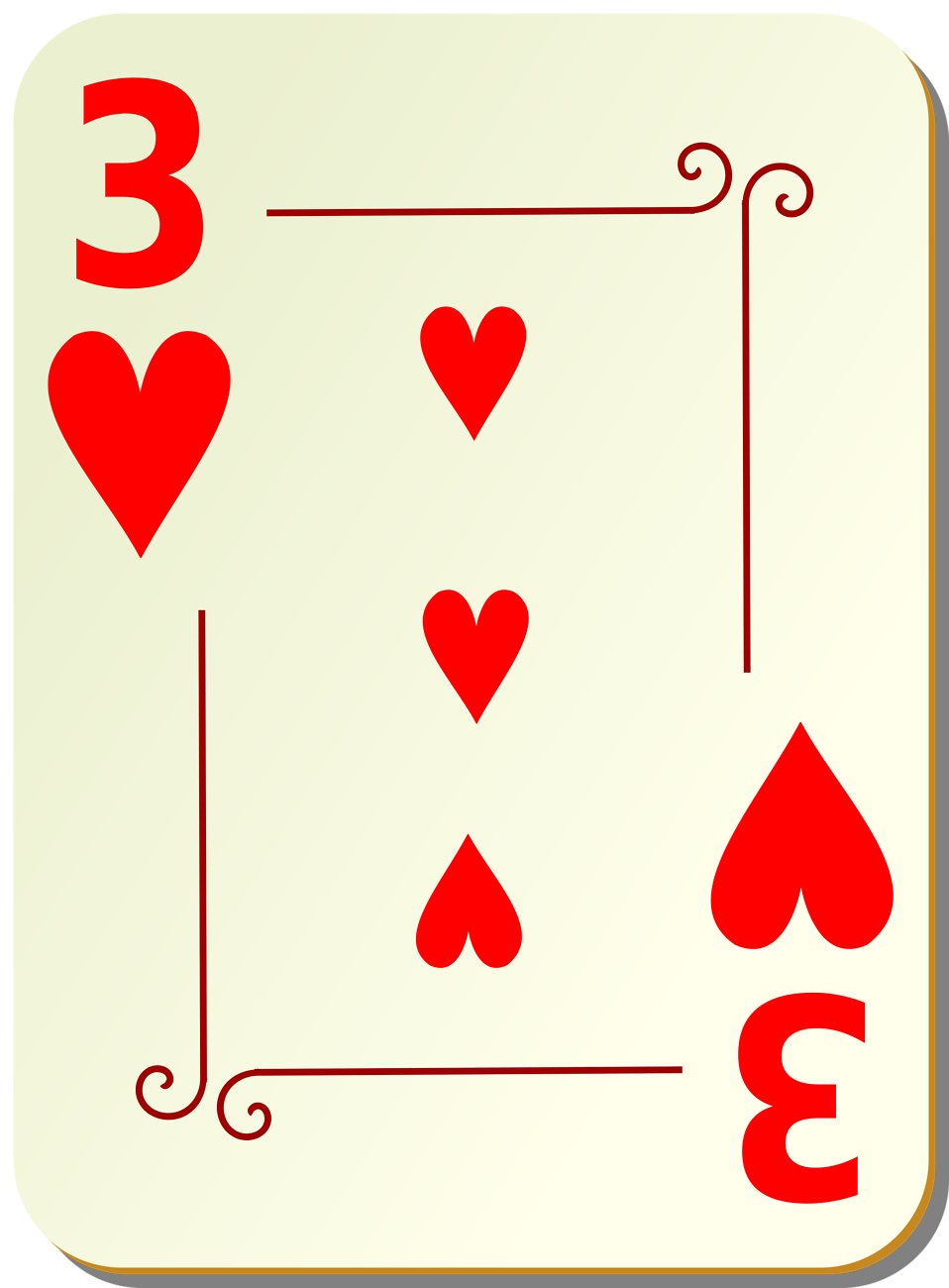## Abstractions

$3+5 = 5+3$

$9+2 = 2+9$ And then $x + y = y + x$

Algebra is a higher level of abstractions

• We have rules that apply to any number. No matter what number

## Clustering

### Teach the computer to think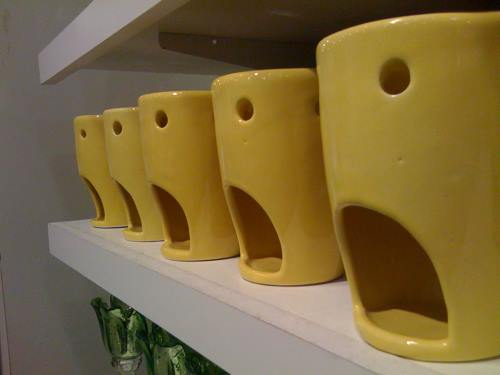## Clustering

Forget differences to find common identity

“New Oxford American Dictionary” defines

cluster |ˈkləstər| noun

• a group of similar objects growing closely together: clusters of grapes.
• a group of people or similar objects positioned or occurring close together: a cluster of antique shops.
• a natural subgroup of a population, used for statistical sampling or analysis.

## Used to…

• split all the samples into meaningful classes
• Find the characteristic of each class
• classify all instances into classes
• determine the class of new instances
• determine the number of classes

## A Correct Clustering

cellular organism; Eukaryota; Metazoa; Bilateria; Coelomata; Deuterostomia; Chordata; Craniata; Vertebrata; Gnathostomata; Teleostomi; Euteleostomi; Sarcopterygii; Tetrapoda; Amniota; Mammalia; Primates; Hominoidea; Hominidae; Homininae; Homo; H.sapiens; Latinamerican; chilean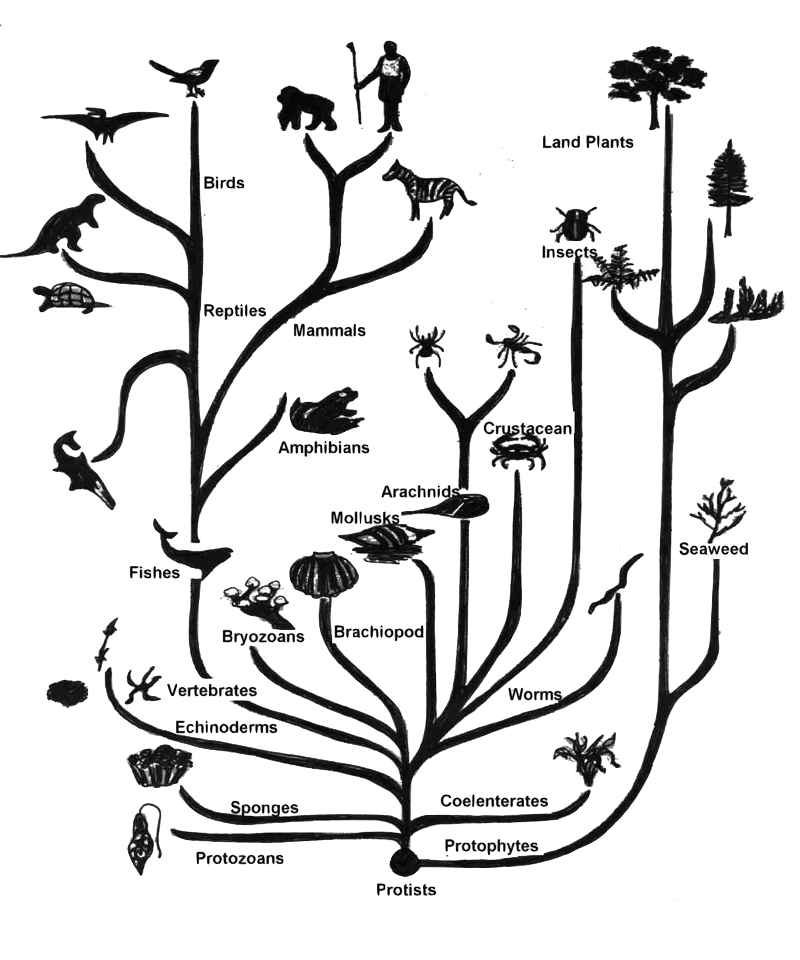Tree of Life

## A CorrectUseful Clustering

• Different groupings can be correct at the same time
• The number of clusters depending on the context
• This is called granularity level
• meaning “the size of the grains”

## Example

• Variables: Gene Expression
• Individuals: cancer samples
• Clustering shows 4 groups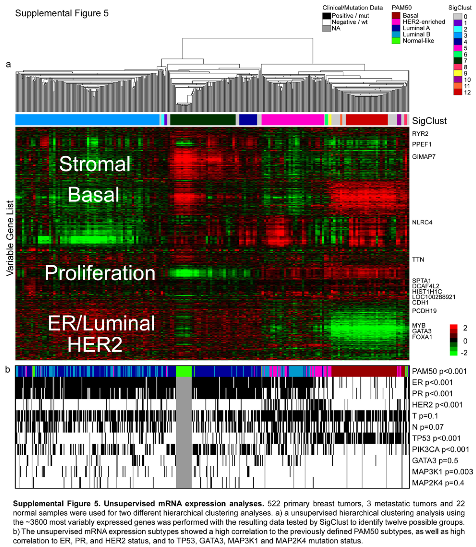## Back to Definition

cluster |ˈkləstər| noun

• a group of similar objects growing closely together

## Distance: a way to measure differences

Let us put a number to measure similarity

• The distance of 2 things is a non-negative number
• smaller distance means more similar
• distance of a thing to itself is zero $\mathrm{dist}(x,x)=0$
• symmetry: $$\mathrm{dist}(x,y)=\mathrm{dist}(y,x)$$
• Triangular inequality $\mathrm{dist}(x,z)\leq\mathrm{dist}(x,y)+\mathrm{dist}(y,z)$

## Example of distance: $$(x-y)^2$$

Here $$x$$,$$y$$,$$z$$ are real numbers, positive or negative.

If $$\mathrm{dist}(x,y)=(x-y)^2$$ then:

• $$\mathrm{dist}(x,y)$$ is never negative
• $$\mathrm{dist}(x,x)=0$$ for any $$x$$
• $$\mathrm{dist}(x,y)=\mathrm{dist}(y,x)$$
• $$\mathrm{dist}(x,z)\leq\mathrm{dist}(x,y)+\mathrm{dist}(y,z)$$

So this is a valid distance

Exercise: prove it

## Hierarchical Clustering

bottom up: joining one by one

• if $$\mathrm{dist}(x, y)$$ is the smallest distance, we join $$x$$ and $$y$$
• we create cluster $$C$$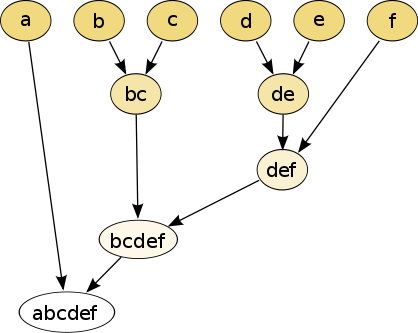## Now we have to measure the distance between elements and clusters

How to measure distance between $$x$$ and $$C$$?

How to measure distance between cluster $$C_1$$ and $$C_2$$?

$\mathrm{dist}(x, C)=\mathrm{mean} (\mathrm{dist}(x, y): y \in C)$ $\mathrm{dist}(C_1, C_2)=\mathrm{mean} (\mathrm{dist}(x, y): x \in C_1, y \in C_2)$ Distance between two clusters is the distance between their mass centers

$\mathrm{dist}(x, C)=\min(\mathrm{dist}(x, y): y \in C)$ $\mathrm{dist}(C_1, C_2)=\min(\mathrm{dist}(x, y): x \in C_1, y \in C_2)$ Distance between two clusters is the smallest distance between their elements

$\mathrm{dist}(x, C)=\max(\mathrm{dist}(x, y): y \in C)$ $\mathrm{dist}(C_1, C_2)=\max(\mathrm{dist}(x, y): x \in C_1, y \in C_2)$ Distance between two clusters is the maximal distance between their elements

## GEO

library(GEOquery)
se <- getGEO(GEO="GSE3541", destdir = "geo-data")

## Accessing expression data

length(se)
 1
se <- se[]
expr <- exprs(se)
pheno <- pData(se)
feature <- fData(se)

## Hierarchical clustering

d <- dist(expr)
tree <- hclust(d, method = "complete")
plot(tree, labels = FALSE)## Euclidean Distance

• square root of the sum of squares
• has a geometrical sense
• “expensive” in computation time

If $$x$$ and $$y$$ are vectors of length $$n$$, then $\mathrm{dist}_2(x,y)=\sqrt{(x_1-y_1)^2+\cdots +(x_n-y_n)^2}$

## Manhattan Distance

Sum of absolute values $\mathrm{dist}_1(x,y)=\vert x_1-y_1\vert +\cdots +\vert x_n-y_n\vert$ Different geometrical meaning

## Why “Manhattan”?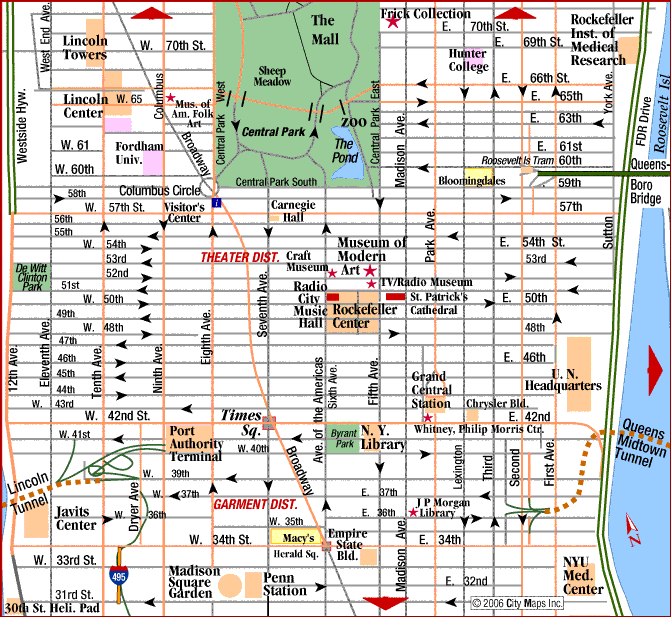## Maximal Distance

$\mathrm{dist}_∞ = max(\vert x_1-y_1\vert ,\ldots,\vert x_n-y_n\vert )$ Only the biggest one matters

## Example

$X = (0,0), Y = (100,1)$ $\mathrm{dist}_1(X,Y) = 101$ $\mathrm{dist}_2(X,Y) = 100.005$ $\mathrm{dist}_\infty(X,Y) = 100$

## Example

$X = (10,1), Y = (100,1)$ $\mathrm{dist}_1(X,Y) = 90$ $\mathrm{dist}_2(X,Y) = 90$ $\mathrm{dist}_\infty(X,Y) = 90$

## For next class

We will start analyzing genomic sequences.

Prepare slides to explain

• FASTA file
• GFF file
• GenBank file

They are explained in Wikipedia and NCBI website.

## Credits of Images

• Chair image by Alex Rio Brazil - Own work, CC BY-SA 3.0, https://commons.wikimedia.org/w/index.php?curid=8045709

• dogs By YellowLabradorLooking_new.jpg: derivative work: Djmirko (talk)YellowLabradorLooking.jpg: User:HabjGolden_Retriever_Sammy.jpg: Pharaoh HoundCockerpoo.jpg: ALMMLonghaired_yorkie.jpg: Ed Garcia from United StatesBoxer_female_brown.jpg: Flickr user boxercabMilù_050.JPG: AleRBeagle1.jpg: TobycatBasset_Hound_600.jpg: ToBNewfoundland_dog_Smoky.jpg: Flickr user DanDee Shotsderivative work: December21st2012Freak (talk) - YellowLabradorLooking_new.jpg Golden_Retriever_Sammy.jpg Cockerpoo.jpg Longhaired_yorkie.jpg Boxer_female_brown.jpg Milù_050.JPGBeagle1.jpgBasset_Hound_600.jpg Newfoundland_dog_Smoky.jpg, CC BY-SA 3.0, https://commons.wikimedia.org/w/index.php?curid=10793219

• allegry of the cave By Veldkamp, Gabriele and Maurer, Markus - Veldkamp, Gabriele. Zukunftsorientierte Gestaltung informationstechnologischer Netzwerke im Hinblick auf die Handlungsfähigkeit des Menschen. Aachener Reihe Mensch und Technik, Band 15, Verlag der Augustinus Buchhandlung, Aachen 1996, Germany, CC BY-SA 3.0, https://commons.wikimedia.org/w/index.php?curid=24826744